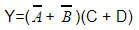August 23, 2023
August 24, 2023
August 23, 2023
###### Operating-Systems
August 24, 2023
 Question 3
Minimum number of NAND gates required to implement the following binary equationA 4 B 5 C 3 D 6
Question 3 Explanation:
AND-OR (or SOP)realization is easily convertible into NAND-NAND realization.
NOT-OR is equivalent to NAND.
Y = (A’+B’) (C+D)
Y = (A’+B’)C + (A’+B’)D
Let X= (A’+B’) , Y= C, and Z= D
One NAND gate is needed for implementing X= (A’ + B’).
Y= XY + XZ
Y= [(XY)’ (XZ)’]’
Three NAND gates are needed for [(XY)’ (XZ)’]’.
Total Four NAND gates are required to implement thY = (A’+B’) (C+D).
Question 3 Explanation:
AND-OR (or SOP)realization is easily convertible into NAND-NAND realization.
NOT-OR is equivalent to NAND.
Y = (A’+B’) (C+D)
Y = (A’+B’)C + (A’+B’)D
Let X= (A’+B’) , Y= C, and Z= D
One NAND gate is needed for implementing X= (A’ + B’).
Y= XY + XZ
Y= [(XY)’ (XZ)’]’
Three NAND gates are needed for [(XY)’ (XZ)’]’.
Total Four NAND gates are required to implement thY = (A’+B’) (C+D).